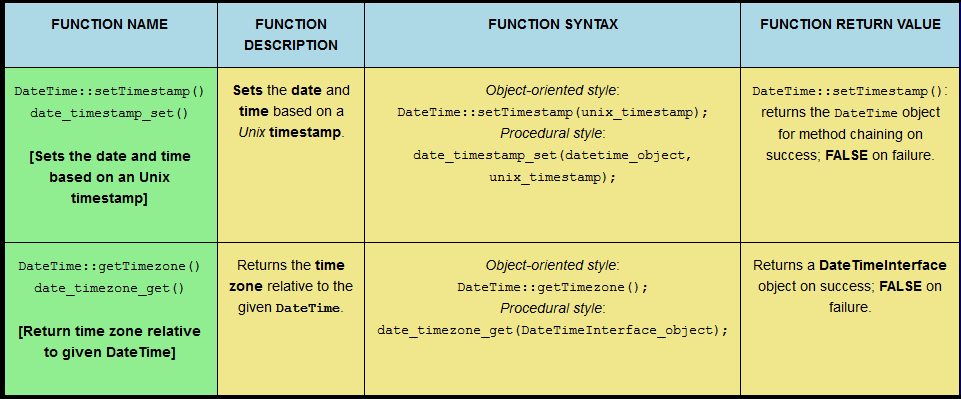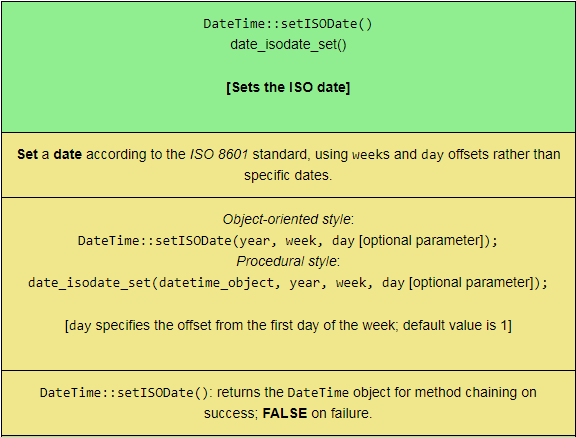# PHP Quiz: All Date/Time FunctionsThis post serves as a reference point for 48 PHP date/time functions. Users can also click a ‘RANDOMIZE’ button, whereupon all the function descriptions, function syntaxes, and function return values are randomized. The user can then rearrange the table cells correctly (thus completing the quiz) or click an ‘AUTO COMPLETE’ button (following further optional manual randomization) and watch the computer complete the quiz itself! The computer completes the quiz at a speed that the human eye can follow, thus aiding learning.

# PHP Date/Time Function Quiz 5: `DateTimeZone::getLocation()`; `timezone_name_from_abbr()`; `DateTimeZone::getName()`; `DateTimeZone::getOffset()`; `DateTimeZone::__construct()`; `DateTimeZone::getTransitions()`; and `timezone_version_get()`.This post serves as a reference point for 7 PHP date/time functions: `DateTimeZone::getLocation()`; `timezone_name_from_abbr()`; `DateTimeZone::getName()`; `DateTimeZone::getOffset()`; `DateTimeZone::__construct()`; `DateTimeZone::getTransitions()`; and `timezone_version_get()`. Users can also click a ‘RANDOMIZE’ button, whereupon all the function descriptions, function syntaxes, and function return values are randomized. The user can then rearrange the table cells correctly (thus completing the quiz) or click an ‘AUTO COMPLETE’ button (following further optional manual randomization) and watch the computer complete the quiz itself! The computer completes the quiz at a speed that the human eye can follow, thus aiding learning.

# PHP Date/Time Function Quiz 4: `microtime()`; `mktime()`; `strftime()`; `strptime()`; `strtotime()`; `time()`; `DateTimeZone::listAbbreviations()`; and `DateTimeZone::listIdentifiers()`.This post serves as a reference point for 8 PHP date/time functions: `microtime()`; `mktime()`; `strftime()`; `strptime()`; `strtotime()`; `time()`; `DateTimeZone::listAbbreviations()`; and `DateTimeZone::listIdentifiers()`. Users can also click a ‘RANDOMIZE’ button, whereupon all the function descriptions, function syntaxes, and function return values are randomized. The user can then rearrange the table cells correctly (thus completing the quiz) or click an ‘AUTO COMPLETE’ button (following further optional manual randomization) and watch the computer complete the quiz itself! The computer completes the quiz at a speed that the human eye can follow, thus aiding learning.

# PHP Date/Time Function Quiz 3: `DateTime::setTimestamp()`; `DateTime::getTimezone()`; `DateTime::setTimezone()`; `date()`; `getdate()`; `gettimeofday()`; `gmdate()`; `gmmktime()`; `gmstrftime()`; `idate()`; and `localtime()`.This post serves as a reference point for 11 PHP date/time functions: `DateTime::setTimestamp()`; `DateTime::getTimezone()`; `DateTime::setTimezone()`; `date()`; `getdate()`; `gettimeofday()`; `gmdate()`; `gmmktime()`; `gmstrftime()`; `idate()`; and `localtime()`. Users can also click a ‘RANDOMIZE’ button, whereupon all the function descriptions, function syntaxes, and function return values are randomized. The user can then rearrange the table cells correctly (thus completing the quiz) or click an ‘AUTO COMPLETE’ button (following further optional manual randomization) and watch the computer complete the quiz itself! The computer completes the quiz at a speed that the human eye can follow, thus aiding learning.

# PHP Date/Time Function Quiz 2: `DateTime::setISODate()`; `DateTime::modify()`; `DateTime::getOffset()`; `date_parse_from_format()`; `date_parse()`; `DateTime::sub()`; `date_sun_info()`; `date_sunrise()`; `date_sunset()`; `DateTime::setTime()`; and `DateTime::getTimestamp()`.This post serves as a reference point for 11 PHP date/time functions: `DateTime::setISODate()`; `DateTime::modify()`; `DateTime::getOffset()`; `date_parse_from_format()`; `date_parse()`; `DateTime::sub()`; `date_sun_info()`; `date_sunrise()`; `date_sunset()`; `DateTime::setTime()`; and `DateTime::getTimestamp()`. Users can also click a ‘RANDOMIZE’ button, whereupon all the function descriptions, function syntaxes, and function return values are randomized. The user can then rearrange the table cells correctly (thus completing the quiz) or click an ‘AUTO COMPLETE’ button (following further optional manual randomization) and watch the computer complete the quiz itself! The computer completes the quiz at a speed that the human eye can follow, thus aiding learning.

# PHP Date/Time Function Quiz: `checkdate()`; `date_add()`; `date_create_from_format()`; `date_date_set()`; `date_default_timezone_get()`; `date_default_timezone_set()`; `date_diff()`; `date_format()`; `date_get_last_errors()`; `DateInterval::createFromDateString()`; and `DateInterval::format()`.This post serves as a reference point for 11 PHP date/time functions: `checkdate()`; `date_add()`; `date_create_from_format()`; `date_date_set()`; `date_default_timezone_get()`; `date_default_timezone_set()`; `date_diff()`; `date_format()`; `date_get_last_errors()`; `DateInterval::createFromDateString()`; and `DateInterval::format()`. Users can also click a ‘RANDOMIZE’ button, whereupon all the function descriptions, function syntaxes, and function return values are randomized. The user can then rearrange the table cells correctly (thus completing the quiz) or click an ‘AUTO COMPLETE’ button (following further optional manual randomization) and watch the computer complete the quiz itself! The computer completes the quiz at a speed that the human eye can follow, thus aiding learning.